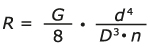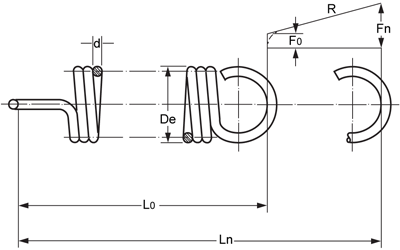# How do you calculate the spring constant of an extension spring?

The spring constant is calculated according to the following formula:R = Spring constant
G = Modulus of shear N/mm2
d4 = wire thickness to the power of 4
D3 = Outside diameter to the power of 3
n = active coils

The spring constant describes how much the force in Newtons is increased per mm the extension spring is drawn out.

The spring constant of an extension spring is linear apart from the introductory section, where the initial tension has to be overcome.

The drawing below shows the increase in force Fn as a result of R.